Whenever we have a lot of cells with formulas, then wanted to change them to permanent values, we need to use the Paste Special functionality of Excel. What if I told you, you can do this in a single click for all formula cells in your selection?

That’s right, Excel Macros can convert formulas into values in one step!

Make sure your Excel has the Developer Tab enabled following this tutorial.

I explain how you can do this below step by step!

What does it do?

Converts formulas into values in your selection

Copy Source Code:

```
Sub ConvertFormulasIntoValues()

Dim rng As Range
Dim formulaCell As Range
Set rng = Selection

'Check each cell in the range if it has a formula
For Each formulaCell In rng
If formulaCell.HasFormula Then
formulaCell.Formula = formulaCell.Value
End If
Next formulaCell

End Sub

```

Final Result: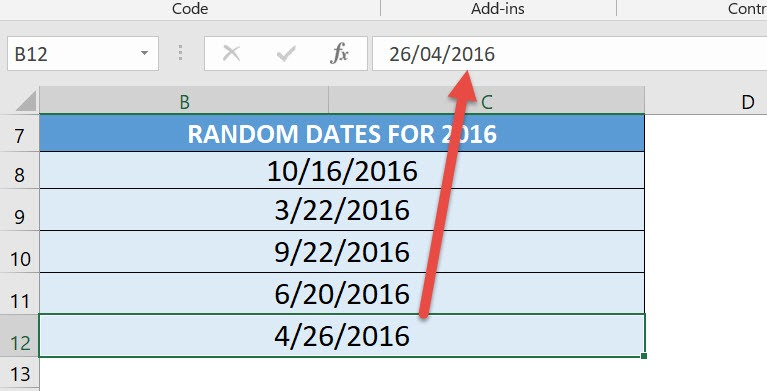Exercise Workbook:Here is our list of formula cells: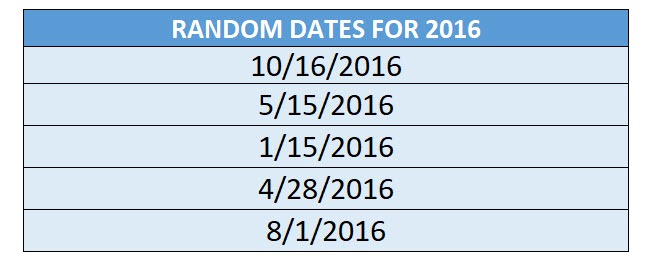To have a better look, you can see it uses the RANDBETWEEN formula. Let us change these to values only!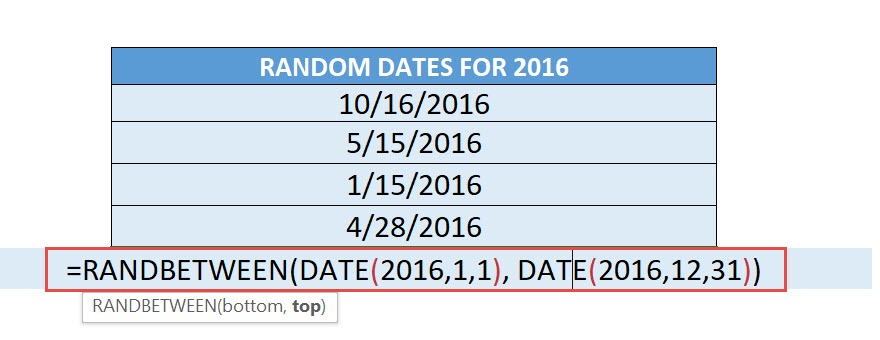STEP 1: Go to Developer > Code > Visual Basic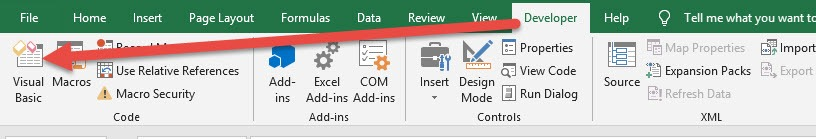STEP 2: Paste in your code and Select Save. Close the window afterwards.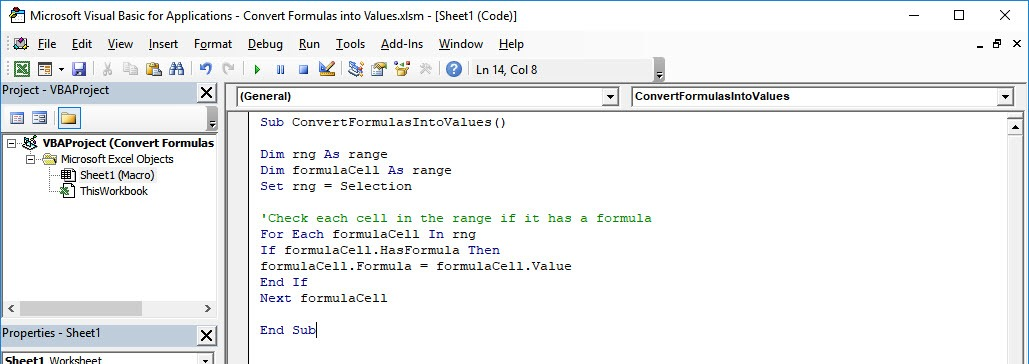STEP 3: Let us test it out!

Open the sheet containing the data. Go to Developer > Code > Macros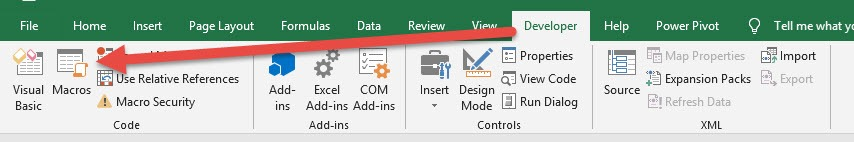Make sure your formula cells and macro are both selected. Click Run.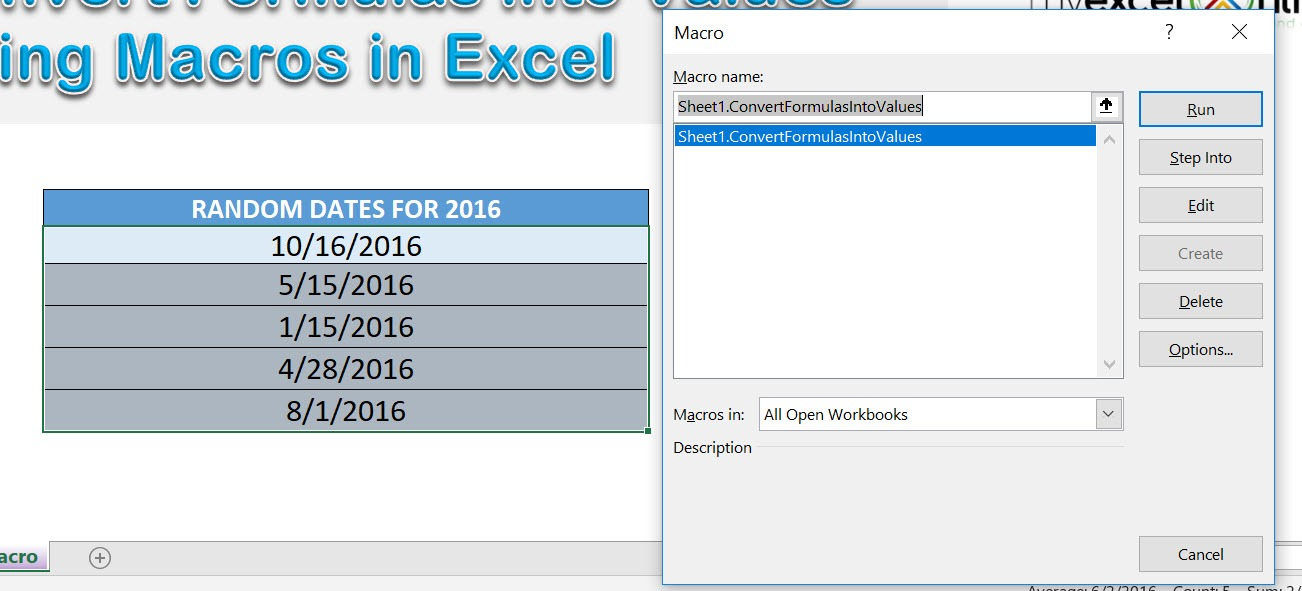With just one click, all of the formula cells are now converted to values! The values also changed because of the nature of the RANDBETWEEN formula, as it changes along with any change done to the workbook 🙂How to Convert Formulas into Values Using Macros In Excel